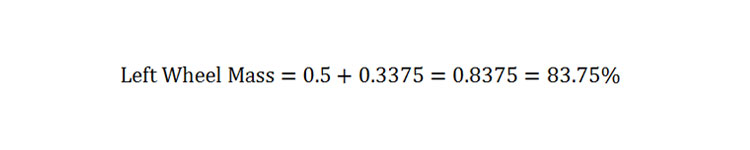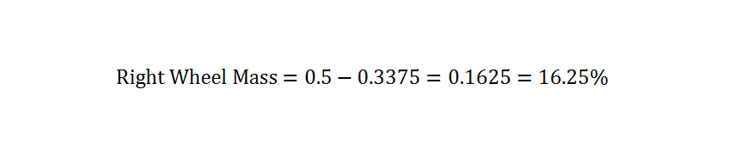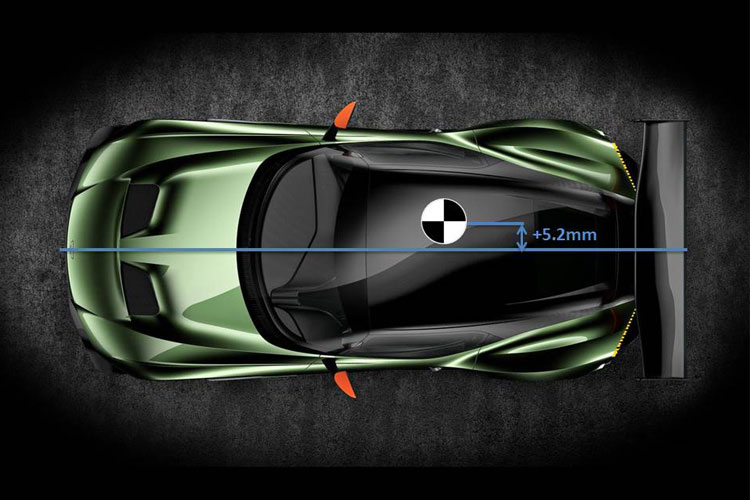# Lateral and Longitudinal Load TransferTwo main types of load transfer exist; lateral load transfer and longitudinal load transfer. In this article we will cover the basic equations for both to provide an understanding of what each is and how it works dynamically.

Lateral load transfer occurs during cornering and is the shift of mass across the wheels due to the centrifugal force and the lateral acceleration. The diagram below shows a typical cornering scenario.When a car is cornering it creates a force called centrifugal force.  This force works against the lateral acceleration which is created by the grip from the tyres known as the tyre cornering forces. The diagram above shows a vehicle cornering around a right hand turn.

In the diagram the cornering forces created by the tyres are what result in the lateral acceleration (ay). The units for “ay” are in m/sec² but for the purposes of the calculations and equation simplifications we need it to be in units of g. Therefore the below equation can be used to convert this.Where “Ay” is the lateral acceleration in g force.Another benefit to having the acceleration in terms of g force is that it can be directly correlated with data logging from sessions out on track that record cornering forces. Therefore if you have any previously logged data or a target g force to generate around a certain corner then that figure could be used directly.

Due to the equationWhere:

• F = Force
• m = Mass
• a = Acceleration

We can relate our scenario to the force equation in terms of the centrifugal force when cornering which is equal to:Where:

• W = Total vehicle mass
• Ay = Lateral acceleration in g

As the centrifugal force is always acting in the opposite direction to the lateral acceleration, the equation for centrifugal force becomes:First of all we must take moments about the inside tyre using the following moment equation:Where:

• WL = Static Mass on Left Wheel (kg)
• t = Track Width (m)
• W = Vehicle Total Mass (kg)
• Ay = Lateral Acceleration in G
• h = Height of centre of gravity (m)

We can simplify and compress this equation to produce:This can be simplified once more to produce the lateral load transfer expressed as a fraction of the total weight of the vehicle:For example, a car cornering at 0.9g with a centre of gravity height of 0.6m and a track width of 1.6m would produce the following:Therefore the lateral load transfer occurring across this car is 33.75% of the total mass of the vehicle.  So assuming the car in question had an equal split of mass on the right and left wheels when stationary this would result in:AndTherefore, a car set up like the above with a static mass of 1000kg now has the following left and right masses when cornering:AndIf the left and right static masses were different to each other then calculate the percentage of the total mass present at each wheel and then subtract and add the LLT percentage as before. The below equation can be used to calculate the mass on each side as a percentage.

If your car has a total mass of 1000kg with 585Kg on the right wheels and 415Kg on the left wheels then:AndThe LLT percentage value can now be subtracted from the right hand percentage and added to the left hand percentage for the right hand corner scenario in this example.

This process does not calculate how the load is distributed across all four wheels but instead shows the total load transferred. The load on individual wheels is dependent on more complex factors and will be covered in the article calculating the ideal spring rates.

When a car is accelerating or braking, a reaction force is generated similar to the centrifugal force generated when cornering.  This reaction force is “WAx”. The longitudinal acceleration is in g force again similar to the lateral load transfer. The g force value is “Ax”. If you have acceleration in meters per second squared then the below equation can be used to convert it into units of g.If the longitudinal acceleration is due to acceleration then it is a positive value. If it is caused by braking then the acceleration should be assigned a negative sign.

The diagram below displays a car accelerating that will be referred to for the initial equation to calculate the load transfer front to rear. It assumes that the centre of gravity position is on the centre line of the track width.Taking moments about the front wheel we can generate the following equation:Where:

• ΔWx = The increase in the rear axle downward load and therefore the decrease in front axle load. Or if braking, it is the decrease in rear axle load and the increase in front axle load in Kg.
• L = The wheel base of the car in meters
• h = The height of the centre of gravity from the ground in meters
• W = Total mass of the car in Kg
• Ax = Longitudinal acceleration in g force.

This equation can be re-organised to produce just the load transfer:So with a centre of gravity height of 0.6m, and wheelbase of 2.7m, a vehicle mass of 1000kg and an acceleration of 1G we get:Therefore we get a transfer of mass of 222.22 Kg from the front wheels to the rear wheels.

In some suspension systems the lateral load transfer can create a large change in ride heights at the front and rear as the car squats under acceleration or dives during braking. The ride height change is due to suspension geometry known as anti-dive, anti-lift and anti-squat geometry.

In the case that your centre of gravity position does not lie exactly in the centre of your track width, the wheels on the same side that the COG is offset to will take a greater proportion of the mass transfer than the other side. In this case we are able to calculate the individual wheel loads at each corner by means of a few simple equations and a co-efficient “Cx”.

The diagram below shows the COG position of a car from the top view with it offset slightly to one side of the car.The offset distance is referred to as e’’. If the COG offset is offset to the right hand side then this number is a positive value; if the COG is offset to the left then this number is a negative value. This is very important for the below calculations. First of all we must create a ratio to use in the below equations known as the offset ratio. If your front and rear track widths are different, then one ratio will need creating for the front wheels and one will need creating for the rear wheels.Where:

• e = Front Offset Ratio
• e’’ = Distance of COG offset from the centre line in meters
• t = Front track width in meters

And:Where:

• e = Rear Offset Ratio
• e’’ = Distance of COG offset from the centre line in meters
• t = Rear track width in meters

The co-efficients “Cx” generated for each wheel now become:The longitudinal load transfer for each wheel can now be calculated using the following equations. In each equation longitudinal acceleration “Ax” is a positive value if the car is accelerating and is negative if the car is braking.Where:

• W1 refers to the front right wheel
• W2 refers to the front left wheel
• W3 refers to the rear right wheel
• W4 refers to the rear left wheel.

### 2 thoughts on “Lateral and Longitudinal Load Transfer”

1.Paulo says:

The right hand side in the last section is for the driver view or a frontal plane view? Thank you, great site!

1.Suspension Secrets says:

Hi Paulo, thanks for your comment. It is from the drivers view from the drivers seat.

Thanks
Suspension Secrets